# please Solve This!! Consider a maximization problem with the optimal tableau in Table 73. The optimal...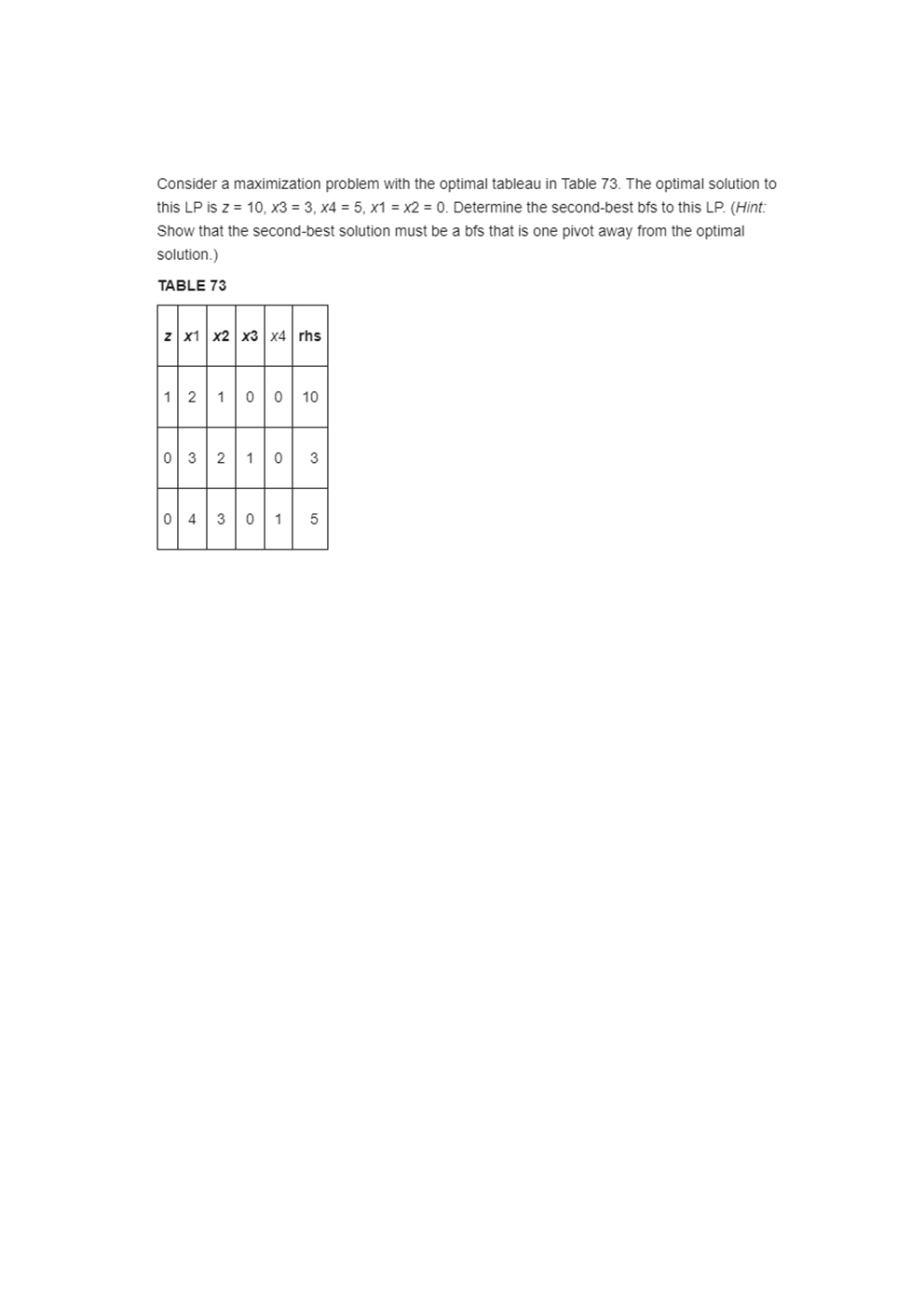Consider a maximization problem with the optimal tableau in Table 73. The optimal solution to this LP is z = 10, x3 = 3, x4 = 5, x1 = x2 = 0. Determine the second-best bfs to this LP. (Hint: Show that the second-best solution must be a bfs that is one pivot away from the optimal solution.) TABLE 73 z X1 X2 X3 X4 rhs 1 2 10 10 10 0 3 2 1 0 3 0 430 11

Sice optimal table is

 Z x1 x2 x3 x4 rhs 1 2 1 0 0 10 0 3 2 1 0 3 0 4 3 0 1 5

Optimal solution is z=10 for x1=x2=0, x3=3,x4=5

To find the second best basic feasible solution i.e.bfs

One pivot away from the optimal solution we choose x2 as the entering variable bcoz it gives the smallest decrease in objective value z and corresponding leaving variable is x3 according to the ratio test

After two iteration new table is determined by applying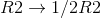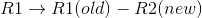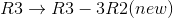z x1 x2 x3 x4 rhs 1 1/2 0 -1/2 0 17/2 0 3/2 1 1/2 0 3/2 0 -1/2 0 -3/2 1 1/2

So second best bfs is x2=3/2 ; x4=1/2 ;z=17/2

#### Earn Coin

Coins can be redeemed for fabulous gifts.

Similar Homework Help Questions
• ### Q4. (Sensitivity Analysis: Adding a new constraint) (3 marks) Consider the following LP max z= 6x1+x2 s.t.xi + x2 S5 2x1 + x2 s6 with the following final optimal Simplex tableau basis x1 r2 S2 rhs 0...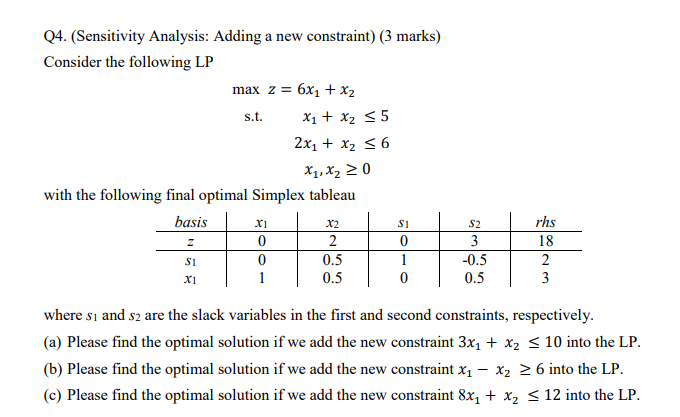Q4. (Sensitivity Analysis: Adding a new constraint) (3 marks) Consider the following LP max z= 6x1+x2 s.t.xi + x2 S5 2x1 + x2 s6 with the following final optimal Simplex tableau basis x1 r2 S2 rhs 0 0 18 0.5 0.5 0.5 0.5 x1 where sı and s2 are the slack variables in the first and second constraints, respectively (a) Please find the optimal solution if we add the new constraint 3x1 + x2 S 10 into the LP (b)...

• ### original right-hand Table Q2 shows the final optimal maximization simplex tableau. The sides were 100 and...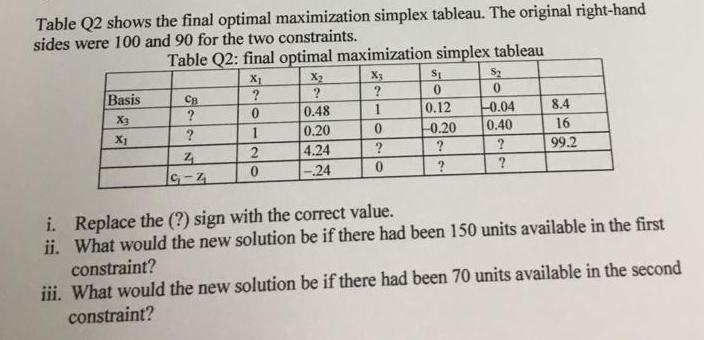original right-hand Table Q2 shows the final optimal maximization simplex tableau. The sides were 100 and 90 for the two constraints. Table Q2: final optimal maximization simplex tableau 0 0.12 8.4 16 ? 99.2 04 0.48 0.20 4.24 -.24 X3 -0.20 0.40 X1 G-2 i. ii. Replace the (?) sign with the correct value. What would the new solution be if there had been 150 units available in the first constraint? ii. What would the new solution be if there...

• ### The following tableau represents a specific simplex iteration for a maximization problem.  &...

The following tableau represents a specific simplex iteration for a maximization problem.            Z   x1   x2   x3   s1   s2   s3 RHS            1   0    a     0   3    5    0   15              0   0    0    1   1    0    b   3            0   1    d     0   1    2    0   5            0   0   -3    0   -2    6    1     c For each part 1)-3) specify any numerical value for each of a, b, c, and d that would:    1) allow x2 to enter the basis to improve the...

• ### Consider the following optimal tableau of a maximization problem where the constraints are of the s...Consider the following optimal tableau of a maximization problem where the constraints are of the s type. (Initial basis consisted of the columns corresponding to the slack variables in order shown) SLACK 0 2 0-2-1/10 2 0 1 01/21/5-1 0 1/2 0 0 0 0 1 1 25-3/10 2 a. Find the optimal objective function value, as well as the value of 0. b. Would the solution be altered if a new activity x, with coefficients (2,0,3) in the constraints,...

• ### Problem #7: Consider the linear program st. max z = 5x, + 3x2 + xz x...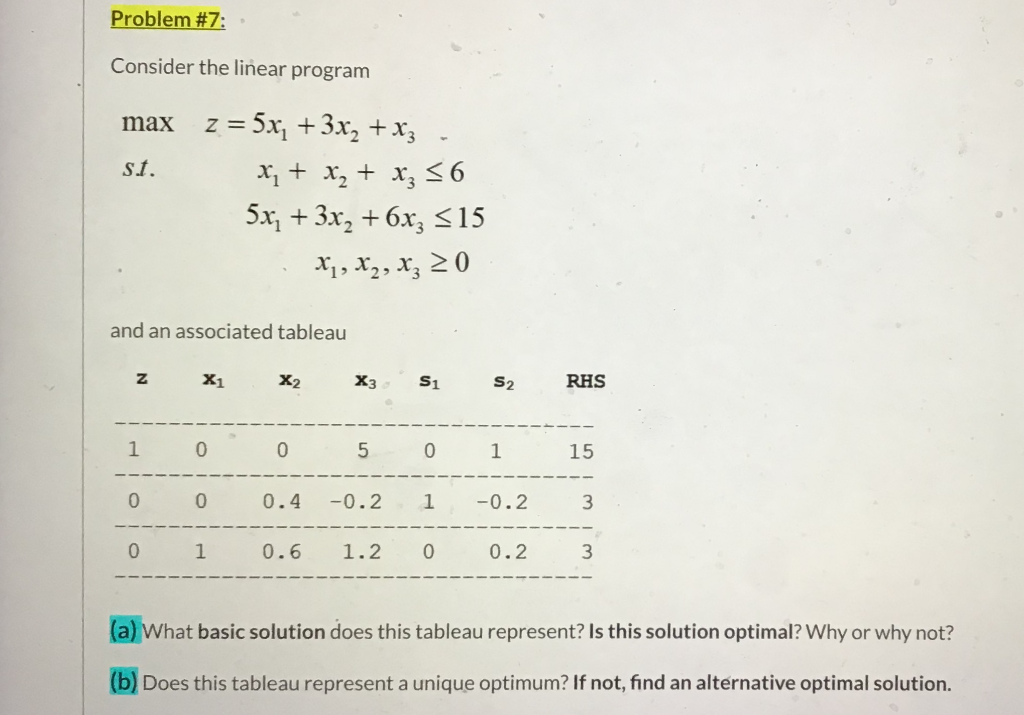Problem #7: Consider the linear program st. max z = 5x, + 3x2 + xz x + x2 + x3 56 5x2 + 3x2 +6x3 =15 X1, X2, xz 20 and an associated tableau N X1 X2 X3 S1 S2 RHS 1 0 0 5 0 1 15 0 0 0.4 -0.2 1 -0.2 3 0 1 0.6 1.2 0 0.2 3 (a) What basic solution does this tableau represent? Is this solution optimal? Why or why not? (b) Does...

• ### Consider the simplex tableau for a maximization problem shown. Provide a particular solution for the given...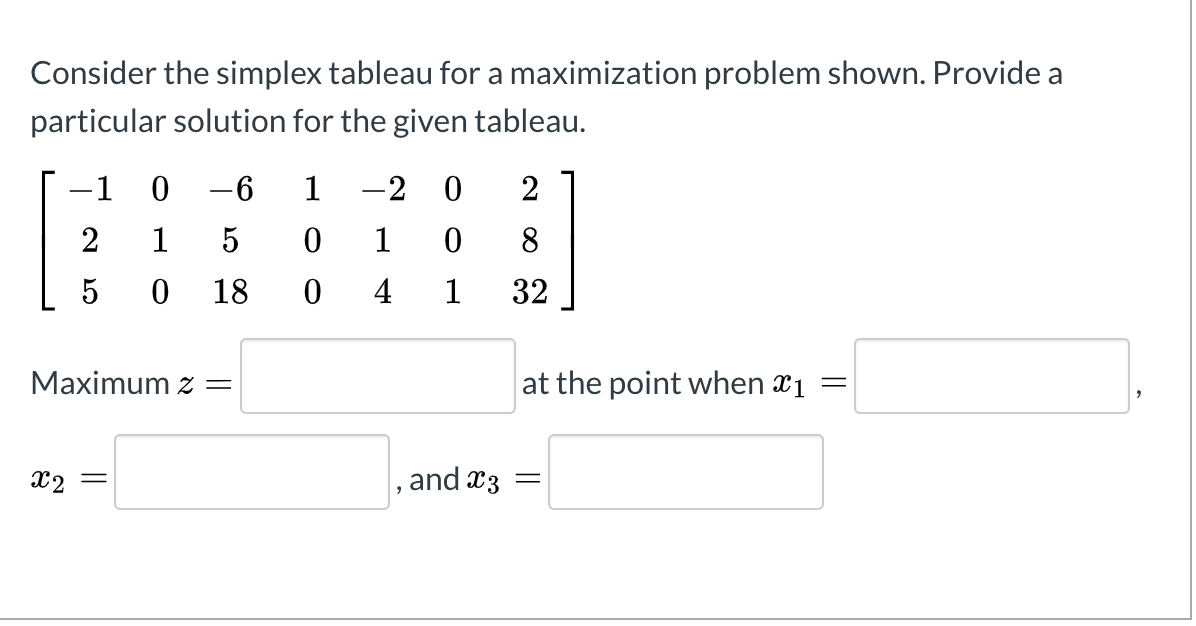Consider the simplex tableau for a maximization problem shown. Provide a particular solution for the given tableau. 1 0 -6 1 -2 0 2 2 1 5 0 0 0 1 4 8 32 5 0 18 1 Maximum z= at the point when Xi = X2 = , and X3 =

• ### Dual problem.

Consider the following LP:max z = -2x1 - x2 + x3s.t.x1 + x2 + x3 ≤ 3x2 + x3 ≥ 2x1+ x3 = 0x1, x2, x3 ≥ 0a) Find the dual of this LP.b) After adding a slack variable s1, subtracting an excess variable e2, and adding artificial variables, a2 and a3, row0 of the LP's optimal tableau is found to bez + 4x1 + e2 + (M-1)a2 + (M+2)a3 = 0Find the optimal solution to the dual of this...

• ### (1 point) Consider the following maximization problem. Maximize P = 9x1 + 7x2 + x3 subject...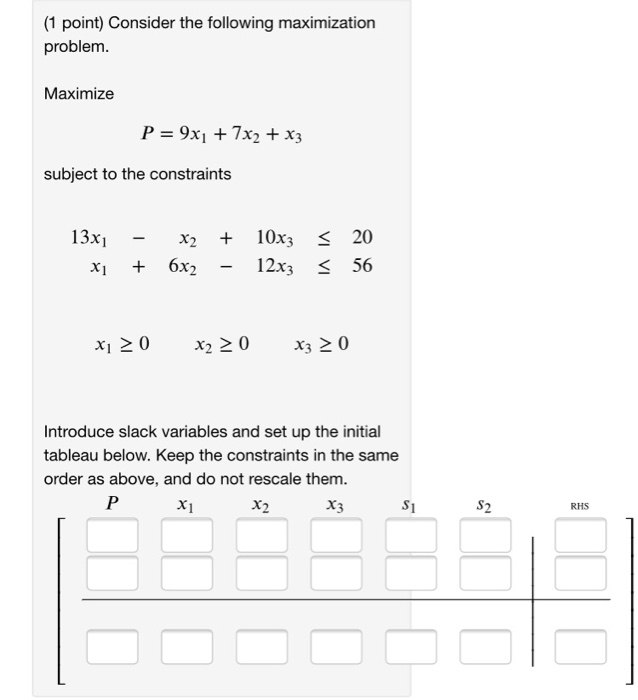(1 point) Consider the following maximization problem. Maximize P = 9x1 + 7x2 + x3 subject to the constraints 13x1 x1 - x2 + 6x2 + - 10x3 12x3 = = 20 56 xi 20 x2 > 0 X3 > 0 Introduce slack variables and set up the initial tableau below. Keep the constraints in the same order as above, and do not rescale them. P X X2 X3 S1 RHS

• ### 1) Consider the simplex tableau obtained after a few iterations: RHS Basic 1 1/4 5/8 57/4 57/4 0 ...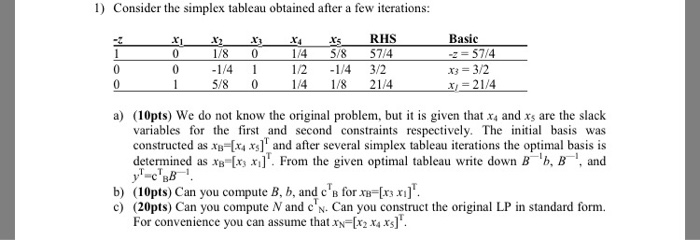1) Consider the simplex tableau obtained after a few iterations: RHS Basic 1 1/4 5/8 57/4 57/4 0 01/4 1 1/8 /2 14 3/2 1/4 1/8 5/8 0 a) (10pts) We do not know the original problem, but is given that x and xs are the slack variables for the first and second constraints respectively. The initial basis was constructed as хв=fu xs] and after several simplex tableau iter tions the optimal basis is determined as x [x, x]. From...

• ### 4) (20 pts) Consider the following optimal Simplex Tableau of an LP problem: 11 12 13...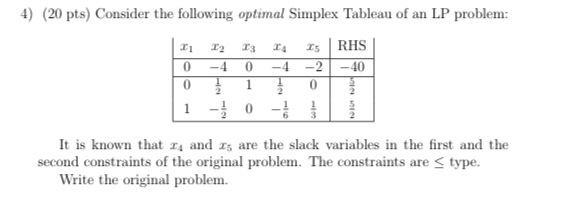4) (20 pts) Consider the following optimal Simplex Tableau of an LP problem: 11 12 13 0 0 0 14 -4 1 RHS -2-40 0 1 1 1 It is known that 14 and 15 are the slack variables in the first and the second constraints of the original problem. The constraints are stype. Write the original problem.